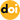### Scheme to Improve the Line Current Distortion of PFC Using a Predictive Control Algorithm

Vol. 15, No. 5, pp. 1168-1177, Sep. 201510.6113/JPE.2015.15.5.1168

###### Abstract

This paper presents a scheme to improve the line current distortion of power factor corrector (PFC) topology at the zero crossing point using a predictive control algorithm in both the continuous conduction mode (CCM) and discontinuous conduction mode (DCM). The line current in single-phase PFC topology is distorted at the zero crossing point of the input AC voltage because of the characteristic of the general proportional integral (PI) current controller. This distortion degrades the line current quality, such as the total harmonic distortion (THD) and the power factor (PF). Given the optimal duty cycle calculated by estimating the next state current in both the CCM and DCM, the proposed predictive control algorithm has a fast dynamic response and accuracy unlike the conventional PI current control method. These advantages of the proposed algorithm lower the line current distortion of PFC topology. The proposed method is verified through PSIM simulations and experimental results with 1.5 kW bridgeless PFC (BLPFC) topology.

###### Statistics
Show / Hide Statistics

Cumulative Counts from September 30th, 2019
Multiple requests among the same browser session are counted as one view. If you mouse over a chart, the values of data points will be shown.

Cite this article

[IEEE Style]

D. J. Kim, J. Park and K. Lee, "Scheme to Improve the Line Current Distortion of PFC Using a Predictive Control Algorithm," Journal of Power Electronics, vol. 15, no. 5, pp. 1168-1177, 2015. DOI: 10.6113/JPE.2015.15.5.1168.

[ACM Style]

Dae Joong Kim, Jin-Hyuk Park, and Kyo-Beum Lee. 2015. Scheme to Improve the Line Current Distortion of PFC Using a Predictive Control Algorithm. Journal of Power Electronics, 15, 5, (2015), 1168-1177. DOI: 10.6113/JPE.2015.15.5.1168.